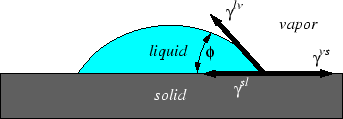Next: Defect Equilibria Up: Continuum Thermodynamics Previous: Electrochemistry

## Interfacial Thermodynamics

A material can store energy at interfaces. The energy contribution is the surface tension multiplied by the area of interface. Surface tension can result in the pressure of two phase being different if the interface between them is curved. The chemical potential at an interface is increased if it is curved, this is the Gibbs-Thompson effect. Material can segregate preferentially to interfaces if it decreases the surface tension. This follows from the Gibbs-Duhem equation for surfaces and the result is called the Gibbs Absorption equation.

Surface tensions act a force balances and specify the contact angle between two phases:Considerations of the geometry of such surfaces has important implications on the sizes of nuclei and therefore on the entire topic of phase transformations.

W. Craig Carter 2002-09-05